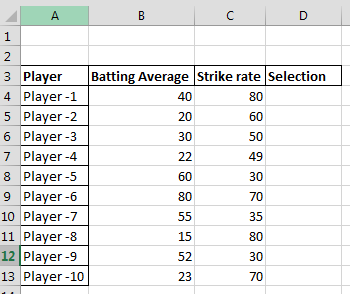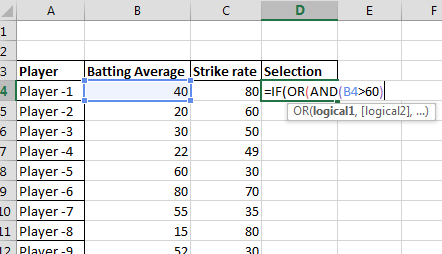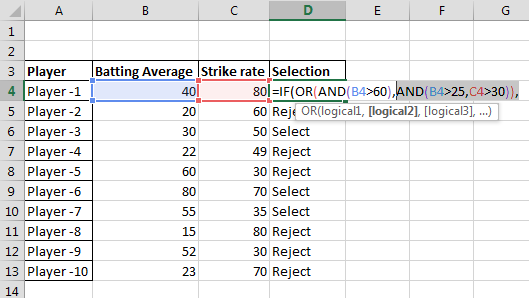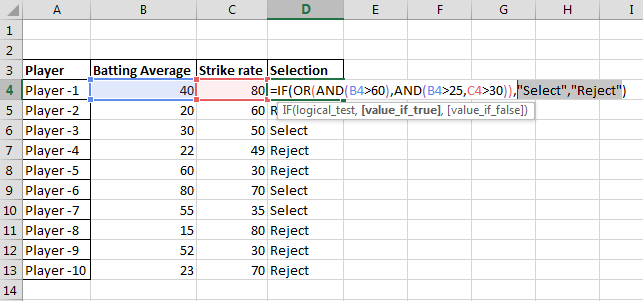#### Nested IF function in Excel

The IF function in Excel allows you to make a logical comparison between a value and what you expect, and returns a result based on whether the comparison is true or false. You can nest multiple IF functions to perform more complex comparisons and make decisions based on multiple conditions.

Let’s together create formula with nested if with combined AND & OR functions. You will need that to select data from your table. This Excel’s lesson is for analytics who would like to select some particular data from the huge data set.

## Nested data preparation

You will input the data and establish a criteria. In the following example, the selection criteria is that players with batting average above 25 and strike rate above 30 or batting average above 60 will be selected.## Inserting nested if formula

In the IF function, you will first insert the OR statement which in this example is batting average should be above 60.

We will enter this in Excel as follows:Now, in the IF function, we will insert the AND statement which in our example is that batting average should be above 25 and strike rate above 30. We will enter this in Excel highlighted as follows:Finally, we will first input what would be the outcome if criteria is fulfilled and then we will enter what will be the outcome if criteria is not fulfilled. In this case, it is Select and Reject.Formula here is:

=IF(OR(AND(B4>60),AND(B4>25,C4>30)),”Select”,”Reject”)

## Using Nested IF Functions to Extract More Complex Data Sets

Nested IF functions can also be used to extract more complex data sets. For example, the following nested IF function can be used to select all players from a batting table who have a batting average above 25, a strike rate above 30, and have played in more than 100 games:

`=IF(OR(AND(B4>60),AND(B4>25,C4>30)),"Select","Reject")`

This formula works by first checking if the player’s batting average is above 60. If it is, then the formula returns Select. If the player’s batting average is not above 60, then the formula checks if the player’s batting average is above 25 and their strike rate is above 30. If both conditions are met, then the formula returns Select. Otherwise, the formula returns Reject.

You can nest as many IF functions as you need, but it’s important to keep in mind that each IF function adds complexity to the formula, so it’s best to use nested IF functions sparingly and only when necessary.